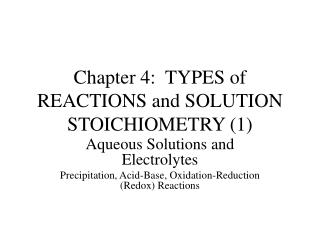DownloadDownload PresentationChapter 4: TYPES of REACTIONS and SOLUTION STOICHIOMETRY (1)

# Chapter 4: TYPES of REACTIONS and SOLUTION STOICHIOMETRY (1)

Download Presentation## Chapter 4: TYPES of REACTIONS and SOLUTION STOICHIOMETRY (1)

- - - - - - - - - - - - - - - - - - - - - - - - - - - E N D - - - - - - - - - - - - - - - - - - - - - - - - - - -
##### Presentation Transcript

1. Chapter 4: TYPES of REACTIONS and SOLUTION STOICHIOMETRY (1) Aqueous Solutions and Electrolytes Precipitation, Acid-Base, Oxidation-Reduction (Redox) Reactions

2. AQUEOUS SOLUTION • Water is the solvent, other species (present in small amounts) are the solutes. • Because water is polar (there is a charge separation between the O and H atoms), it has a very high capacity to dissolve cmps. • When ionic cmps (or salts) dissolve in water, a solution of ions forms. • These ions are cations(aq) and anions(aq).

3. Figure 4.1 The Water Molecule is POLAR (note net partial charges on O and H)

4. Fig 4.2 Polar H2O Molecules Interact with the + and - Ions of a Salt Assisting in the Dissolving Process (hydration)

5. AQUEOUS SOLUTIONS (2) • Electrolytes are cmps that form ions in water and, therefore, conduct electricity. • The extent of ionization (solubility) varies from cmp to cmp leading to strong (>70% ionization; many ions in soln) and weak (<5%; few dissolved ions) electrolytes. • KF(aq)  K+(aq) F-(aq) ~ 100% ionization • AgF(aq)  Ag+(aq) F-(aq) ~ 0% ionization

6. AQUEOUS SOLUTIONS (3) • Fig 4.4 shows relationship between electrolyte strength and light bulb current. • Water can also dissolve nonionic cmps, especially those that are polar. (ethanol) • Finally, many nonpolar molecules dissolve in water but do not produce ions in water; these are nonelectrolytes (sucrose, pure water).

7. STRONG ELECTROLYTES • Three classes of strong electrolytes (~100% solubility in water) • soluble salts (T4.1) • strong acids (HCl, HBr, HI, H2SO4, HNO3, HClO4); in general, acids produce H+(aq) and anion in water: HBr(aq)  H+(aq) + Br-(aq) (Arrhenius definition) • strong bases (M1AOH, M2A(OH)2 for M2A= Ca, Ba, Sr); in general, bases produce OH-(aq) and cation in water: KOH(aq)  K+(aq) + OH-(aq) (Arrhenius) • Memorize T4.1 and 6 strong acids and 8 strong bases.

8. WEAK ELECTROLYTES • Three classes of weak electrolytes (< 5% solubility) • insoluble salts (T4.1) • weak acids (acids other than those listed as strong). When weak acids dissolve in water, few H+(aq) are produced. HF(aq)  H+(aq) + F-(aq) • weak bases (bases other than those listed as strong) When weak bases dissolve in water, few OH-(aq) are produced. Fe(OH)2(aq)  Fe2+(aq) + 2OH-(aq)

9. QUANTIFYING SOLUTION COMPOSITION (MOLARITY, M) • Concentration: Measure of quantity of solute in solvent • Molarity = M = #mol solute per l liter soln • # mol solute = molarity * volume soln (L) = M * V • # mol ion in a soln • Dilution of concentrated (c, stock) soln to make dilute (d) soln: Mc * Vc = Md * Vd

10. Another Look at Strong Electrolytes • When Na3PO4(s) is added to water, it dissolves completely (see T4.1). • How do we express the resulting solution? • Na3PO4(aq)  3Na+(aq) + PO43-(aq) • One mol salt yield 4 mols of dissolved ions in soln. (Ch 3 stoichiometry) • 3[PO43-(aq)] = [Na+(aq)]

11. Figure 4.12a-c A Measuring Pipet is Used to Add Acetic Solution to a Volumetric Flask

12. SOLUTION STOIOCHIOMETRY • Stoichiometric calculations for reactions taking place in aqueous solutions require • Balanced chem eqn Amount of chemicals present in rxn • VA, MA  [#mol A  #mol B]  VB if MA and MB are known • [ * ] above designates basic stoich conversion from Chapter 3

13. Problems • Chapter 4: 20, 22, 24, 30, 32